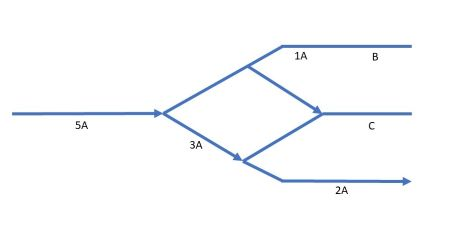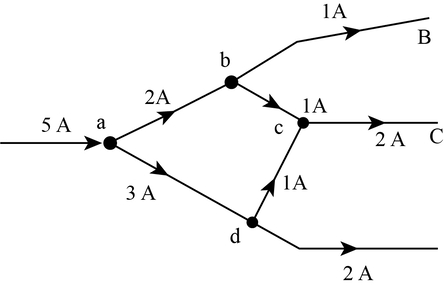# In the given figure, the current through the different segments of the wire is shown. Find the...

## Question:

In the given figure, the current through the different segments of the wire is shown. Find the magnitude and direction of the current in the wire through segments B and C.## Kirchhoff's Current Law

Kirchhoff's Current Law or KCL is very useful in solving any circuits while doing network analysis. This law defines that the total sum of all the currents from various branches of the circuit entering a particular node of that circuit is equal to the total sum of the current leaving out from that node to various branches of the circuit; hence it can be concluded that a net current in that node will be zero.

From KCL, we know that:

{eq}\sum {{I_{in}} = \sum {{I_{out}}} } {/eq}

Here, {eq}{I_{in}} {/eq} is current entering the node.

• {eq}{I_{out}} {/eq} is the current leaving the node.

The diagram of the above problem is shown below:In the above problem, we can see that the 5A current is entering the node. According to KCL, we can write that 2A and 3A current will reach node b and d respectively, again at node b if we apply KCl, 1A current will go to node c, Another 1 A current will go to wire B as shown in the diagram, again if we apply KCl at node d, 3A incoming current at node d within which 1 A goes to node c, and 2A goes to the third wire as shown in the diagram now if we apply KCL at node c we get 2A current entering the wire C as shown in the figure.Parallel Circuits: Definition & Examples

from

Chapter 3 / Lesson 10
10K

In this lesson, learn more about parallel electrical circuits. We'll review some electrical circuit fundamentals, look at the defining characteristics of parallel circuits, and explore several concrete examples.